# [转载图文] Java 运算符

742主题 10精华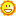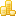学币
5065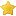荣耀
14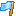rank
123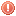违规
0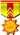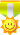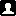发表于 2020-5-7 21:42:42 | 显示全部楼层 |阅读模式
 Java 运算符　　计算机的最基本用途之一就是执行数学运算，作为一门计算机语言，Java也提供了一套丰富的运算符来操纵变量。我们可以把运算符分成以下几组： 算术运算符关系运算符位运算符逻辑运算符赋值运算符其他运算符 算术运算符　　算术运算符用在数学表达式中，它们的作用和在数学中的作用一样。下表列出了所有的算术运算符。 　　表格中的实例假设整数变量A的值为10，变量B的值为20： 操作符描述例子+加法 - 相加运算符两侧的值A + B 等于 30-减法 - 左操作数减去右操作数A – B 等于 -10*乘法 - 相乘操作符两侧的值A * B等于200/除法 - 左操作数除以右操作数B / A等于2％取余 - 左操作数除以右操作数的余数B%A等于0++自增: 操作数的值增加1B++ 或 ++B 等于 21（区别详见下文）--自减: 操作数的值减少1B-- 或 --B 等于 19（区别详见下文）实例　　下面的简单示例程序演示了算术运算符。复制并粘贴下面的 Java 程序并保存为 Test.java 文件，然后编译并运行这个程序： 实例 public>　　运行实例 » 　　以上实例编译运行结果如下： 　　a + b = 30 　　a - b = -10 　　a * b = 200 　　b / a = 2 　　b % a = 0 　　c % a = 5 　　a++   = 10 　　a--   = 11 　　d++   = 25 　　++d   = 27 　　 自增自减运算符　　1、自增（++）自减（--）运算符是一种特殊的算术运算符，在算术运算符中需要两个操作数来进行运算，而自增自减运算符是一个操作数。 实例 public>　　运行结果为： 　　进行自增运算后的值等于4 　　进行自减运算后的值等于2 　　解析： 　　int b = ++a; 拆分运算过程为: a=a+1=4; b=a=4, 最后结果为b=4,a=4 　　int d = --c; 拆分运算过程为: c=c-1=2; d=c=2, 最后结果为d=2,c=2 　　2、前缀自增自减法(++a,--a): 先进行自增或者自减运算，再进行表达式运算。 　　3、后缀自增自减法(a++,a--): 先进行表达式运算，再进行自增或者自减运算实例： 实例 public>　　运行结果为： 　　自增运算符前缀运算后a=6，x=12 　　自增运算符后缀运算后b=6，y=10 关系运算符　　下表为Java支持的关系运算符 　　表格中的实例整数变量A的值为10，变量B的值为20： 运算符描述例子==检查如果两个操作数的值是否相等，如果相等则条件为真。（A == B）为假。!=检查如果两个操作数的值是否相等，如果值不相等则条件为真。(A != B) 为真。> 检查左操作数的值是否大于右操作数的值，如果是那么条件为真。（A> B）为假。< 检查左操作数的值是否小于右操作数的值，如果是那么条件为真。（A =检查左操作数的值是否大于或等于右操作数的值，如果是那么条件为真。（A> = B）为假。<=检查左操作数的值是否小于或等于右操作数的值，如果是那么条件为真。（A <= B）为真。实例　　下面的简单示例程序演示了关系运算符。复制并粘贴下面的Java程序并保存为Test.java文件，然后编译并运行这个程序： Test.java 文件代码： public>　　以上实例编译运行结果如下： 　　a == b = false 　　a != b = true 　　a > b = false 　　a < b = true 　　b >= a = true 　　b <= a = false 　　 位运算符　　Java定义了位运算符，应用于整数类型(int)，长整型(long)，短整型(short)，字符型(char)，和字节型(byte)等类型。 　　位运算符作用在所有的位上，并且按位运算。假设a = 60，b = 13;它们的二进制格式表示将如下： 　　A = 0011 1100 　　B = 0000 1101 　　----------------- 　　A&B = 0000 1100 　　A | B = 0011 1101 　　A ^ B = 0011 0001 　　~A= 1100 0011 　　 　　下表列出了位运算符的基本运算，假设整数变量 A 的值为 60 和变量 B 的值为 13： 操作符描述例子＆如果相对应位都是1，则结果为1，否则为0（A＆B），得到12，即0000 1100|如果相对应位都是 0，则结果为 0，否则为 1（A | B）得到61，即 0011 1101^如果相对应位值相同，则结果为0，否则为1（A ^ B）得到49，即 0011 0001〜按位取反运算符翻转操作数的每一位，即0变成1，1变成0。（〜A）得到-61，即1100 0011<< 按位左移运算符。左操作数按位左移右操作数指定的位数。A << 2得到240，即 1111 0000>> 按位右移运算符。左操作数按位右移右操作数指定的位数。A >> 2得到15即 1111>>> 按位右移补零操作符。左操作数的值按右操作数指定的位数右移，移动得到的空位以零填充。A>>>2得到15即0000 1111实例　　下面的简单示例程序演示了位运算符。复制并粘贴下面的Java程序并保存为Test.java文件，然后编译并运行这个程序： Test.java 文件代码： public>　　以上实例编译运行结果如下： 　　a & b = 12 　　a | b = 61 　　a ^ b = 49 　　~a = -61 　　a << 2 = 240 　　a >> 2  = 15 　　a >>> 2 = 15 　　 逻辑运算符　　下表列出了逻辑运算符的基本运算，假设布尔变量A为真，变量B为假 操作符描述例子&&称为逻辑与运算符。当且仅当两个操作数都为真，条件才为真。（A && B）为假。| |称为逻辑或操作符。如果任何两个操作数任何一个为真，条件为真。（A | | B）为真。！称为逻辑非运算符。用来反转操作数的逻辑状态。如果条件为true，则逻辑非运算符将得到false。！（A && B）为真。实例　　下面的简单示例程序演示了逻辑运算符。复制并粘贴下面的Java程序并保存为Test.java文件，然后编译并运行这个程序： 实例 public>　　以上实例编译运行结果如下： 　　a && b = false 　　a || b = true 　　!(a && b) = true 　　 短路逻辑运算符　　当使用与逻辑运算符时，在两个操作数都为true时，结果才为true，但是当得到第一个操作为false时，其结果就必定是false，这时候就不会再判断第二个操作了。 实例 public>　　运行结果为： 使用短路逻辑运算符的结果为false　　a的结果为5 　　解析：该程序使用到了短路逻辑运算符(&&)，首先判断 a 赋值运算符　　下面是Java语言支持的赋值运算符： 操作符描述例子=简单的赋值运算符，将右操作数的值赋给左侧操作数C = A + B将把A + B得到的值赋给C+ =加和赋值操作符，它把左操作数和右操作数相加赋值给左操作数C + = A等价于C = C + A- =减和赋值操作符，它把左操作数和右操作数相减赋值给左操作数C - = A等价于C = C - A* =乘和赋值操作符，它把左操作数和右操作数相乘赋值给左操作数C * = A等价于C = C * A/ =除和赋值操作符，它把左操作数和右操作数相除赋值给左操作数C / = A，C 与 A 同类型时等价于 C = C / A（％）=取模和赋值操作符，它把左操作数和右操作数取模后赋值给左操作数C％= A等价于C = C％A<< =左移位赋值运算符C << = 2等价于C = C << 2>> =右移位赋值运算符C >> = 2等价于C = C >> 2＆=按位与赋值运算符C＆= 2等价于C = C＆2^ =按位异或赋值操作符C ^ = 2等价于C = C ^ 2| =按位或赋值操作符C | = 2等价于C = C | 2实例　　面的简单示例程序演示了赋值运算符。复制并粘贴下面的Java程序并保存为Test.java文件，然后编译并运行这个程序： Test.java 文件代码： public>　　以上实例编译运行结果如下： 　　c = a + b = 30 　　c += a  = 40 　　c -= a = 30 　　c *= a = 300 　　c /= a = 1 　　c %= a  = 5 　　c <<= 2 = 20 　　c >>= 2 = 5 　　c >>= 2 = 1 　　c &= a  = 0 　　c ^= a   = 10 　　c |= a   = 10 　　 条件运算符（?:）　　条件运算符也被称为三元运算符。该运算符有3个操作数，并且需要判断布尔表达式的值。该运算符的主要是决定哪个值应该赋值给变量。 　　variable x = (expression) ? value if true : value if false 　　 实例Test.java 文件代码： public>　　以上实例编译运行结果如下： 　　Value of b is : 30 　　Value of b is : 20 　　 instanceof 运算符　　该运算符用于操作对象实例，检查该对象是否是一个特定类型（类类型或接口类型）。 　　instanceof运算符使用格式如下： 　　( Object reference variable ) instanceof  (class/interface type) 　　 　　如果运算符左侧变量所指的对象，是操作符右侧类或接口(class/interface)的一个对象，那么结果为真。 　　下面是一个例子： 　　String name = "James"; 　　boolean result = name instanceof String; // 由于 name 是 String 类型，所以返回真 　　 　　如果被比较的对象兼容于右侧类型,该运算符仍然返回true。 　　看下面的例子： class Vehicle {}public>　　以上实例编译运行结果如下： trueJava运算符优先级　　当多个运算符出现在一个表达式中，谁先谁后呢？这就涉及到运算符的优先级别的问题。在一个多运算符的表达式中，运算符优先级不同会导致最后得出的结果差别甚大。 　　例如，（1+3）＋（3+2）*2，这个表达式如果按加号最优先计算，答案就是 18，如果按照乘号最优先，答案则是 14。 　　再如，x = 7 + 3 * 2;这里x得到13，而不是20，因为乘法运算符比加法运算符有较高的优先级，所以先计算3 * 2得到6，然后再加7。 　　下表中具有最高优先级的运算符在的表的最上面，最低优先级的在表的底部。 类别 操作符关联性 后缀() [] . (点操作符)左到右一元++ -- + - ～ ！从右到左乘性 * /％左到右加性 + -左到右移位 >> >>>  << 左到右关系 >  >=  <  <= 左到右相等 ==  !=左到右按位与＆左到右按位异或^左到右按位或|左到右逻辑与&&左到右逻辑或| |左到右条件？：从右到左赋值= + = - = * = / =％= >> = << =＆= ^ = | =从右到左逗号，左到右

1.如果您喜欢这篇帖子，请给作者点赞评分，点赞会增加帖子的热度，评分会给作者加学币。(评分不会扣掉您的积分，系统每天都会重置您的评分额度)。
2.回复帖子不仅是对作者的最好奖励，还可以获得学币奖励，请尊重作者的劳动成果，拒绝做伸手党！
3.发广告、灌水回复等违规行为一经发现直接禁言，如果本帖内容涉嫌违规，请点击论坛底部的举报反馈按钮，也可以在【投诉建议】板块发帖举报。